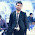### Max-Min problem by divide n conquer (An efficient approach)

//WAP to solve max-min problem using divide n conquer

#include
#include
#define max 20

void main()
{
int a[max],n,i,j,max1,min,temp;
clrscr();
cout<<"\nEnter no. of elements:";
cin>>n;
cout<<"\nEnter "<
for(i=0;i
{
cin>>a[i];
}

i=0; j=n-1;

while(i
{
if(a[i]>a[j])
{
temp=a[i];
a[i]=a[j];
a[j]=temp;
}
i++;
j--;
}

min=a;
max1=a[n/2];

for(i=0;i<(n/2);i++)
{
if(a[i]
{
min=a[i];
}
}

for(i=(n/2);i
{
if(a[i]>max1)
{
max1=a[i];
}
}

cout<<"\nMax="<
cout<<"\nMin="<

getch();
}

1.good blog. For explanation with time complexity & Algorithm this link also help:
http://somnathkayal.blogspot.in/2012/08/finding-maximum-and-minimum-using.html

1.yeah....
if u want to know i can tell you by calculating complexities.